# How To Estimate A Horse's Weight

It's useful to know how much a horse weighs for such things as calculating feed rations, knowing how much de-wormer to administer, and more.

While it's not always practical to find a scale big enough to accommodate a horse, there are several ways commonly used to estimate a horse's weight.

Below: This horse's weight on a livestock scale was 1,320 pounds.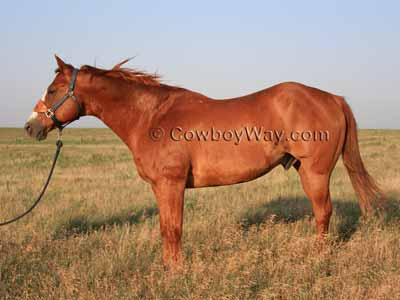In the article below we describe how we used three different horses to test two common methods of estimating horse weight: 1) by using a height/weight tape and 2) by performing a common weight calculation. Then, we compared the estimated weights to actual weights on a livestock scale.

## Height / Weight Tapes

Probably the most common method people use to estimate their horse's weight these days is to use a. To use one of these tapes you simply put the tape around the horse's girth (aka "heart girth") and read the estimated weight on the tape.

It's might be interesting to know there are several different makers of height/weight tapes, and the estimates they provide can vary. Usually the estimates are similar, but actually knowing which tape is most accurate for your horse can be difficult unless you can also weigh your horse on a livestock scale and compare results.

## Weight  Calculation

Another way to estimate a horse's weight is to use a "regular" tape measure - the kind that measures in inches - to measure a horse's girth and length. Then, you perform the following calculation:

Heart girth X heart girth X length, divided by 330, + 50 = weight.

For example:
If a horse's heart girth was 78" and his length was 65", the calculation would be:

78 X 78 X 65 ÷ 330 + 50 = 1,248 pounds, approximate weight.

How to take the measurements for the weight calculation:

### Measuring Heart Girth

To measure a horse's heart girth, measure (in inches) from the base of the withers down to a couple of inches behind the horse's front legs, under the belly, then up the opposite side to where you started. Notice this will cause your tape measure to run at an angle as shown by the blue line in the photograph below. The measurement you arrive at is your horse's heart girth.### Measuring Body Length

To measure a horse's length, measure (in inches) from the point of the shoulder to the point of the rump. Notice this may cause your tape measure to run at an angle as shown by the blue line in the photograph below. The measurement you arrive at is your horse's length.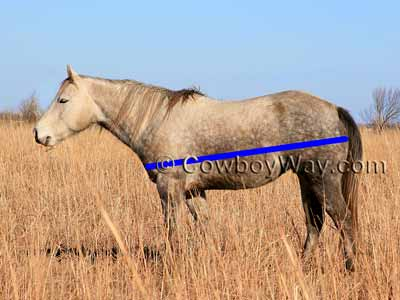#### Estimated Weights Vs. Actual Weights

The three horses below were measured, and their estimated weight was calculated, according to the illustrations and instructions above. Then we used a height/weight tape to see what it gave as an estimated weight. Lastly, we weighed them on a livestock scale to compare their actual weights to their estimated weights. This was all done on the same day within about a 1 1/2 hour time span.

Horse #1

15:1 hand mare.

Heart girth: 77.5 inches
Length: 68 inches

Estimated weights:

• Weight calculation: 1,288 pounds
• Height/weight tape: 1,187 pounds

Actual weight on a livestock scale: 1,320 pounds.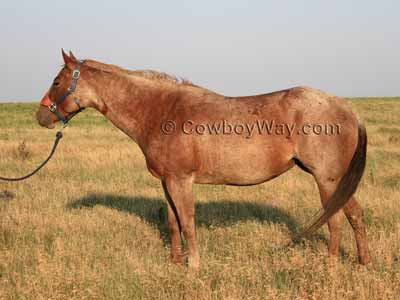Horse #2

15 hand gelding.

Heart girth: 76.5 inches
Length: 68 inches long

Estimated weights:

• Weight calculation: 1,256 pounds
• Height/weight tape: 1,187 pounds

Actual weight on a livestock scale: 1,320 pounds.Horse #3

15 hand gelding.

Heart girth: 78 inches
Length: 65 inches long

Estimated weights:

• Weight calculation: 1,248 pounds
• Height/weight tape: 1,172 pounds

Actual weight on a livestock scale: 1,330 pounds.#### The Results

Horse #1

Weight Calculation
1,320 Actual weight
1,288 Weight calculation estimate
32 Pounds difference

Height/Weight Tape
1,320 Actual weight
1,187 Height/weight tape estimate
133 Pounds difference

Horse #2

Weight Calculation
1,320 Actual weight
1,256 Weight calculation estimate
64 Pounds difference

Height/Weight Tape
1,320 Actual weight
1,187 Height/weight tape estimate
133 Pounds difference

Horse #3

Weight Calculation
1,330 Actual weight
1,248 Weight calculation estimate
82 Pounds difference

Height/Weight Tape
1,330 Actual weight
1,172 Height/weight tape estimate
158 Pounds difference

#### In Conclusion

In our experiment neither the height/weight tape nor the weight calculation estimated the horses' weights with total accuracy compared to their weights on a scale.

Between the and measuring for the calculation, the height/weight tape was far easier to use. It was very simple for one person to put the height/weight tape around the horses' heart girth and read the estimated weight. In repeated use, we seemed to naturally put the height/weight tape in the same place every time and therefore received consistent weight estimates.

Measuring the horses' length for the weight calculation, though, was not quite as easy. It wasn't exactly difficult, but it was easiest to do with two people instead of one. You also had to be careful to place the tape measure at the same start and end points in order to consistently come up with the same measurement. In our experiment changing the length measurement by one inch (longer or shorter) resulted in an approximate difference in the weight estimate of about 20 pounds (heavier or lighter).

So......we slightly prefer the height/weight tape to the weight calculation. Neither method was totally accurate, but it was much easier to come up with consistent results with the height/weight tape. "Weighing" a horse over time with a height/weight tape may not give 100% accurate results, but it can give a reasonable comparison of relative weights for a horse, that is, you at least know approximately how much weight the horse is gaining or losing.

#### Notes

Height/Weight Tape: To use the height/weight tape we ran it around the horses' heart girth from the base of the withers to just behind the front legs. To the best of our knowledge this is the correct, and most common, way of using a height/weight tape. However, we do know people who say that the correct way to use the tape is to put it at the top of the horse's withers, not at the base. We did that, too, just for fun, and it increased each horse's estimated weight by 50 to 60 pounds, which was still less than their scale weights.

Alternate Method Of Measuring Length: Another common way of measuring a horse's length to estimate weight using the calculation given above is to measure from the center of the chest, along the horse's side, to the center of the tail (instead of measuring from the point of the shoulder to the point of the hip like the instructions above). We measured this way, too, and it increased each horse's weight to be heavier than their scale weights by 100 - 176 pounds.

Certified Scales: Weight scales, like weight estimates, are rarely 100% accurate. However, using reasonably reliable scales is one way to try to get the most accurate results. The we used to weigh the horses for this article were at a livestock auction facility that has their scales certified for accuracy according to state law.

CowboyWay.com

Some images and/or other content on this website are copyright © their respective owners.

Horse Tips
Simple, every day tips for cowboys, cowgirls,
and horse lovers.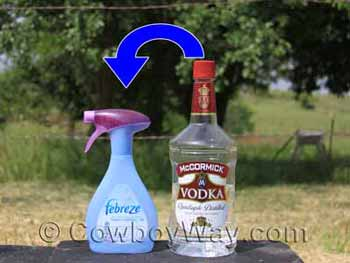Carpenter Photos
Artwork from the owners of CowboyWay.com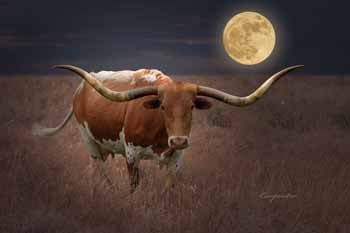Trending